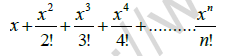# CBSE Class 11 Computer Science Question Paper Set N Solved

Read and download CBSE Class 11 Computer Science Question Paper Set N Solved designed as per the latest question paper pattern and Class 11 examination guidelines issued by CBSE, NCERT and KVS. The past year Question Papers for Class 11 Computer Science have been provided with solutions which will help students to assess their performance and find out topics in Computer Science grade 11 which they need to improve to get better marks in Standard 11 exams. After solving these last year papers also refer to solved Sample Papers for Class 11 Computer Science available on our website to build strong understanding of the subject

## Computer Science Question Paper Class 11

Students can refer to the below Class 11 Computer Science Question Paper designed to help students understand the pattern of questions that will be asked in Grade 11 exams. Please download CBSE Class 11 Computer Science Question Paper Set N Solved

### Computer Science Class 11 Question Paper

1. a. What is the difference between CISC and RISC Computers?

b. What is a Cache memory? Why it is considered crucial for a microprocessors performance?

c. What is the difference between SRAM and DRAM?

d. What are the basic characteristics of a Microprocessor?

e. Explain Serial Port.

f. What do you mean by Bluetooth? Explain.

g. Explain i) USB Port   ii) AGP Port

h. Write a note on:

i) Blu Ray Disc   ii) CD‟s

2.a. What is the importance of main ( ) function in C++?

b. Explain the various ways of giving comments in C++.

c. Explain the following terms with an example:

i) Token  ii) Run-time error

3. a. What do you mean by Dynamic initialization of a variable? Give an example.

b. What do you mean by Access modifier const. Explain with an example.

c. What are the advantages and disadvantages of floating-point numbers over integers?

4. a. What do you mean by implicit and explicit type conversion?

b. Consider the following code snippet:

if(a>b)
c=a;
else
c=b;
Convert this into equivalent C++ code using conditional operator.

c. Differentiate between = and == in C++. Explain with example.

d. Construct logical expressions to represent the following conditions:
i) ch is an uppercase character
ii) weight is greater than or equal to 115 but less than 125

e. Write the output of the following:

i) int t, y, s=3;
y=(t=4, t+s);
cout<< s * t <<‟\t‟<<y;

ii) int p=50;
int s =++p + 20;
int t = p-- + 30;
cout<< ”S=” <<s << ”\n” << ”T=” <<t;

f. Write the corresponding C++ expressions for the following mathematical expressions:
i) | ex cosx |

ii)5.a. What is “fall-through”? What is the significance of default clause in a switch statement?

b. What is an Empty loop? Give an example.

c. How are entry-controlled loops different from exit-controlled loops?

d. Rewrite the following code using do…while loop:

for(int p=25; p<=500; p+=25)
cout<< p << “\t”;
cout<<“end of loop”;

e. Predict the output of the following C++ program:

#include<iostream.h>
void main( )
{ char n1,n2;
for(n1=‟H‟; n1>=‟A‟; n1-= 2)
{ for(n2=‟A‟; n2<=n1; n2++)
cout<<n2;
cout<<endl;
}}

f. Rewrite the following program after removing the syntactical error(s), if any. Underline each correction.

#include<iostream.h>
Void main{ }
{ int i=1; s=1;
for(; i<5; s*=i, ++i)
cout>>s;
}

g. Rewrite the following code fragment using switch-case statement:

char code;
cin >> code;
if(code == „S‟)
cout<<“Summer ”;
else if(code == „W‟)
cout<<“ Winter ”;
else if(code == „R‟)
cout<<“ Rainy ”;
else
cout<<“Enter correct code”;

6. a. Write a program to input three integers and print the largest of the three. 3

b. Write a C++ program to generate the following pattern:
1
12
1 2 3
1 2 3 4

c. Write a program to display the following series:
1 4 7 10 …………….40

d. Write a program to input an integer and print the multiplication table of it till 10.

For example: If the number accepted is 5, the table should be printed in the format
5 * 1 = 5
5 * 2 = 10 …. Upto 5 * 10 = 50.

7. a. Write a program to accept monthly salary from the user. Find and display income tax with the help of following rules:

Monthly Salary                Income Tax

9000 or more           40% of monthly salary
7500 - 8999             30% of monthly salary
7499 or less             20% of monthly salary

1. To reverse an integer
2. Check Odd or Even
3. Quit

The program reads a choice (1,2 or 3) from the user and perform the following:
If choice is 1, read an integer and print the number in the reversed form.(eg. If number is 567, output is 765).
If choice is 2, reads a number and check whether it is odd or even.
If choice is 3, then the program should terminate.
Any other choice should display an error message.
The menu should keep on displaying till the user wants it.

c. Write a program to accept an integer and check whether it is a prime number or not and display proper message as output.

d. Write a program to accept the value of x and n and display the sum of the following series: